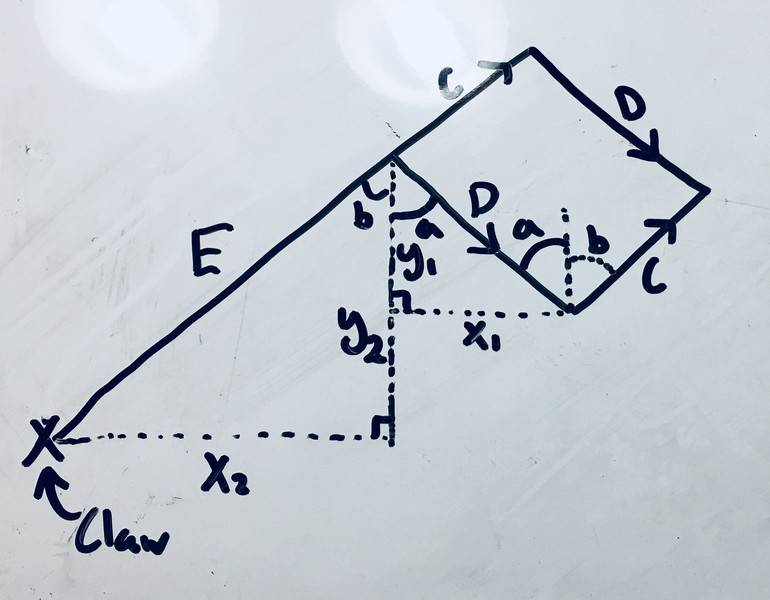# Help creating a coordinate system for a robotic arm

• I
• Dreezy
IanIn summary, the conversation discusses the difficulty in determining the necessary servo angles to align a robotic arm's claw to a given coordinate. The solution involves calculating a set of functions and using a matrix to determine the precise movements of each servo. The end goal is to optimize the path for the arm's movement.

#### Dreezy

Hey guys, I would appreciate some help with the math behind creating a working coordinate system for a robotic arm. I am currently trying to determine what servo angles are necessary to align a robotic arm's claw to the given coordinates. Geometrically simplified, the robotic arm is a parallelogram with two servos at one vertex and one side extended further for the claw. Here is a diagram, pardon my drawing skills:The sides marked D are parallel and equivalent, as are the sides marked C. E is of fixed length and is attached to C (in reality it is just the extension of the C piece). The servos at bottom vertex DC adjust angles a and b to move sides D and C from the vertical. The claw itself is at the end of E, and x refers to the horizontal distance and y the vertical distance from the origin of the two angles a and b (AKA where the servos are placed).

As for what I have tried, I have calculated the claw's coordinates to be X=D*sin(a)+E*sin(b) and Y=D*cos(a)-E*cos(b), but I am unable to solve the system of equations such that I can determine what to set angles a and b to move the claw to a given point. When using substitution, I only end up with an extremely convoluted equation that my calculator cannot parse, leading me to believe I am going down the wrong path. Any help would be much appreciated!
(Also would like to mention for full transparency that this was cross posted to the Math Stack Exchange to no avail)

#### Attachments

##D\sin a=X-E\sin b##
##D\cos a=Y-E\cos b##
Square both sides and add the equations to eliminate ##a##. This leaves you with an equation of the form ##C_1\sin b+C_2\cos b+C_3=0##. Can you solve it?

•andrewkirk
Long ago (1975) I had the same problem. I'll sketch the problem and solution here:

1. Assume that you have a robot arm with 6 servos and 6 position encoders. Name the output of the encoders M1..M6 (machine coordinates).
2. Assume that you want to position the robot arm + tool at the position (x, y, z) with tool orientation (φ, θ, ψ).
3. Also assume that you have a set of continuously differentiable functions f1..f6 such that x=f1(M1..M6) etc.
4. Decide on a δ>0 which shall be used in further calculations.
5. For each Mi, calculate Δfij=(fj(M1..Mi+δ,..M6)-f1(M1..M6).
6. You now have all the elements in a 6x6 matrix A[i, j] which is an approximation of the derivative of the 6-dimensional function F(M, X) (machine coordinates to Euclidean coordinates)
7. Calculate the inverse matrix A-1
8. Calculate a small step in the direction you want the arm and tool to move - call it $\Delta\vec{ X}$. Now you know that $\Delta\vec{ M}\approx A^{-1}\Delta \vec{X}$ which gives you the amount every servo should move. Move there.
9. Calculate the exact position from the machine coordinates.
10. If you are not at the end position, go to 5.

Last edited:
•berkeman
Thanks Svein for sharing your experience, I was not at all expecting someone on these forums to have dealt with such a thing in the past! Your explanation seems a bit above my math ability at the moment, but I am most certainly going to save this and come back to it when I can better understand it.
kuruman said:
##D\sin a=X-E\sin b##
##D\cos a=Y-E\cos b##
Square both sides and add the equations to eliminate ##a##. This leaves you with an equation of the form ##C_1\sin b+C_2\cos b+C_3=0##. Can you solve it?
@kuruman I have tried to follow along (though I have made ##E\cos(b)## positive to match my problem) and have ended up with ##(-2x*E)\sin(b)+(2y*E)\cos(b)+(x^2+y^2-D^2+E^2)=0##, but I unfortunately do not know where to go from here. Perhaps I am unaware of some identity or I have just not been following along properly. Thank you for your assistance!

This is of the form ##C_1\sin b+C_2 \cos b+C_3=0## where the meaning of the ##C_i## constants is obvious.
Let ##u=\sin b## in which case ##\cos b = \sqrt{1-u^2}##. Substitute and you will have a quadratic in ##u## to solve.

In 6D space there are many paths from initial to final position. Once you have figured this out you can optimise the path for least energy or least time or any other feature of the path taken.

Cheers